Definitions of Square Dance Calls and Concepts
Breaker {anything} [C3A]Index -->  Plus  |  A1  |  A2  |  C1  |  C2  |  C3A  |  C3B  |  C4  |  NOL  |
Definitions (Text Only) -->  Plus  |  A1  |  A2  |  C1  |  C2  |  C3A  |  C3B  |  C4  |  NOL  |
 Find call:

 \$B8@8l(BColumns, Magic Columns, \$B\$^\$?\$OE,@Z\$J(B T-Bone \$B\$K\$J\$C\$F\$\$\$k(B 2 x 4 \$B\$+\$i!%(B

Ends \$B\$O(B Cast Off 3/4 \$B\$r(B, Centers \$B\$O(B Box Counter Rotate 1/4 \$B\$r\$7\$F(B 1/2 Box Circulate \$B\$r\$7\$^\$9!%(B \$B\$=\$7\$F(B, Center Line \$B\$,(B anything call (\$B\$b\$7(B anything call \$B\$,(B 1, 2, 3 \$B\$N\$h\$&\$J?t\$@\$C\$?\$i(B, 1/4 \$B\$K\$=\$N?t\$r3]\$1\$?(B Cast Off 1/4, Cast Off 2/4\$B!J(B1/2\$B!K(B, Cast Off 3/4) \$B\$r\$7(B, \$BB>\$N?M\$O(B move up (Phantom Hourglass Circulate) \$B\$r\$7\$^\$9!%(B

anything call \$B\$K\$h\$j\$^\$9\$,(B, \$BIaDL(B Parallel Lines, Twin Diamonds \$B\$^\$?\$O(B Hourglass \$B\$G=*\$o\$^\$9!%(B

Breaker Mix: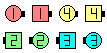Breaker Mix\$B\$NA0(B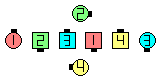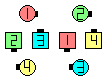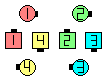Outsides Cast Off 3/4 as Centers Box Counter Rotate 1/4 \$B\$H(B 1/2 Box Circulate\$B\$N8e(B Outside Diamond \$B\$N?M\$,(B'move up' \$B\$N8e(B \$B\$3\$3\$,(B Breaker Nothing.Center Line \$B\$N?M\$,(B anything call \$B\$r\$9\$kI,MW\$,\$"\$j\$^\$9(B Centers Mix\$B\$N8e(B (\$B=*\$o\$j(B)

\$BCm

• \$B;O\$a\$N%Q!<%H\$N8e(B, outside dancers (\$B>e\$N?^\$G(B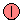,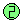,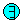,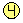) \$B\$O(B move up \$B\$r\$7(B, anything call \$B\$K\$O2C\$o\$i\$J\$\$\$3\$H\$rK:\$l\$J\$\$\$G\$/\$@\$5\$\$!%(B
• Breaker 3 \$B\$,0lHVIaDL\$N;H\$\$J}\$G(B, Linear Action \$B\$^\$?\$O(B Tally-Ho \$B\$K\$H\$F\$b46\$8\$,;w\$F\$\$\$^\$9!%(B
•CALLERLAB definition for Breaker AnythingChoreography for Breaker anythingComments? Questions? Suggestions?